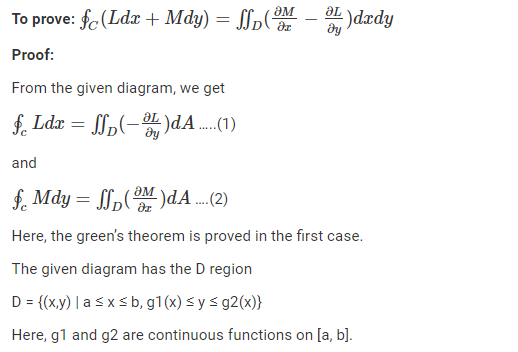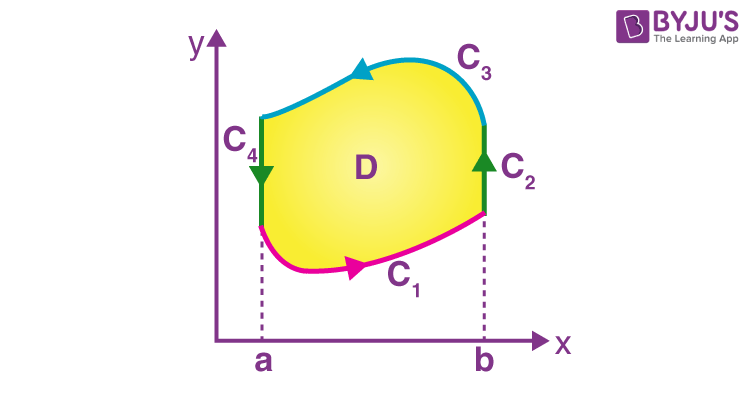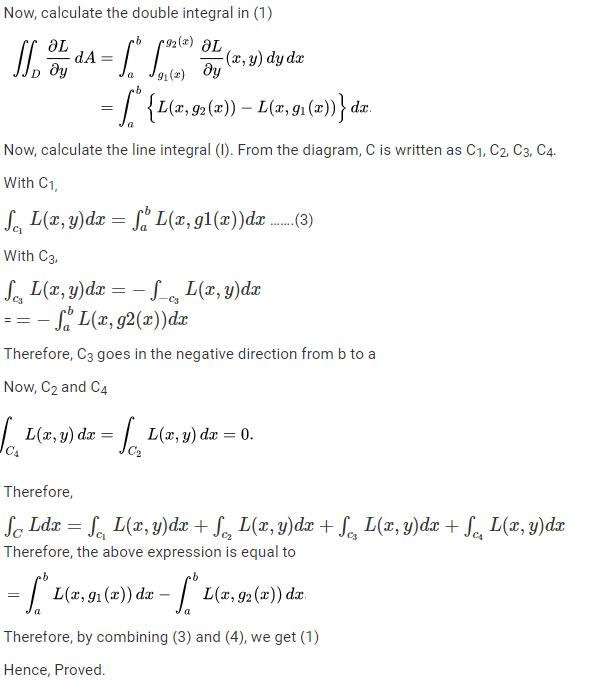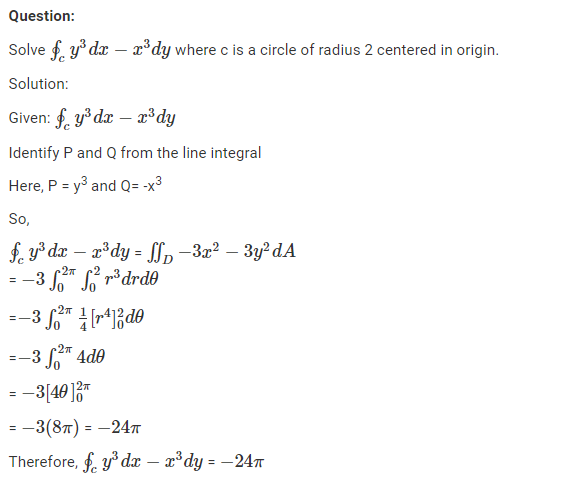# Green’s Theorem

Green’s theorem is mainly used for the integration of the line combined with a curved plane. This theorem shows the relationship between a line integral and a surface integral. It is related to many theorems such as Gauss theorem, Stokes theorem.

Green’s theorem is used to integrate the derivatives in a particular plane. If a line integral is given, it is converted into a surface integral or the double integral or vice versa using this theorem. In this article, you are going to learn what is Green’s Theorem, its statement, proof, formula, applications and examples in detail.

## What is Green’s Theorem?

Green’s theorem is one of the four fundamental theorems of calculus, in which all of four are closely related to each other. Once you learn about the concept of the line integral and surface integral, you will come to know how Stokes theorem is based on the principle of linking the macroscopic and microscopic circulations. Similarly, Green’s theorem defines the relationship between the macroscopic circulation of curve C and the sum of the microscopic circulation that is inside the curve C.## Green’s Theorem Statement

Let C be the positively oriented, smooth, and simple closed curve in a plane, and D be the region bounded by the C. If L and M are the functions of (x, y) defined on the open region, containing D and have continuous partial derivatives, then the Green’s theorem is stated as

$$\begin{array}{l}\oint_{C}(Ldx+Mdy)= \iint_{D}(\frac{\partial M}{\partial x}-\frac{\partial L}{\partial y})dxdy\end{array}$$

Where the path integral is traversed counterclockwise along with C.

## Green’s Theorem Proof

The proof of Green’s theorem is given here. As per the statement, L and M are the functions of (x, y) defined on the open region, containing D and having continuous partial derivatives. So based on this we need to prove:## Green’s Theorem Area

With the help of Green’s theorem, it is possible to find the area of the closed curves.From Green’s theorem,

$$\begin{array}{l}\oint_{C}(Ldx+Mdy)= \iint_{D}(\frac{\partial M}{\partial x}-\frac{\partial L}{\partial y})dxdy\end{array}$$

If in the formula,

$$\begin{array}{l}(\frac{\partial M}{\partial x}-\frac{\partial L}{\partial y})= 1,\end{array}$$

Then we have,

$$\begin{array}{l}\oint_{C}(Ldx+Mdy)= \iint_{D}dxdy\end{array}$$

Therefore, the line integral defined by Green’s theorem gives the area of the closed curve. Therefore, we can write the area formulas as:

• $$\begin{array}{l}A = -\int_{c}ydx\end{array}$$
• $$\begin{array}{l}A = \int_{c}xdy\end{array}$$
• $$\begin{array}{l}A = \frac{1}{2}\int_{c}(xdy-ydx)\end{array}$$

## Green Gauss Theorem

If Σ is the surface Z which is equal to the function f(x, y) over the region R and the Σ lies in V, then

• $$\begin{array}{l}\iint_{\sum }P(x, y, z)d\sum \ exists.\end{array}$$
• $$\begin{array}{l}\iint_{\sum }P(x, y, z)d\sum =\iint_{R}P(x, y, f(x,y))\sqrt{1+f_{1}^{2}(x,y)+f_{2}^{2}(x,y)}ds\end{array}$$

It reduces the surface integral to an ordinary double integral.

Green’s Gauss theorem can be stated from the above expression.

If P(x, y, z), Q(x, y, z), and R((x, y, z) are the three points on V, and it is bounded by the region Σ* and α, β, and γ are the direction angles, then

$$\begin{array}{l}\int \iint_{V}[P_{1}(x, y,z)+Q_{2}+R_{3}(x, y, z)]dV=\iint_{\sum^{\ast }}[P(x, y, z)cos\alpha + Q(x, y, z)cos\beta +R(x, y, z)cos\gamma ]d\sum\end{array}$$

### Green’s Theorem Example

Let us solve an example based on Green’s theorem.### Green’s Theorem Applications

Green’s Theorem is the particular case of Stokes Theorem in which the surface lies entirely in the plane. But with simpler forms. Particularly in a vector field in the plane. Also, it is used to calculate the area; the tangent vector to the boundary is rotated 90° in a clockwise direction to become the outward-pointing normal vector to derive Green’s Theorem’s divergence form.

### Green’s Theorem Problems

1. Using Green’s formula, evaluate the line integral ∮C(x-y)dx + (x+y)dy, where C is the circle x2 + y2 = a2.
2. Calculate ∮C -x2y dx + xy2dy, where C is the circle of radius 2 centered on the origin.
3. Use Green’s Theorem to compute the area of the ellipse (x2/a2) + (y2/b2) = 1 with a line integral.

For more Maths-related theorems and examples, download BYJU’S – The Learning App and also watch engaging videos to learn with ease.